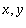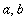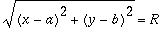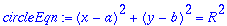Four ways to plot a circle

Plotting a circle is quite straightforward, and also unexciting. However, since it is so familiar an object, it is probably useful to use it to compare four different methods for doing the same thing.

First, let's recall that a circle can be expressed as the set of points () which are distancefrom the center (). That is,or, sinceis always positive, we can square both sides to obtain the usual equation

> circleEqn:= (x-a)^2+(y-b)^2=R^2;1. As a pair of functions

2. In polar coordinates

3. In parametric form

4. Described implicitly

5. By cheating